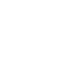Research in Pairs
Research Community
Elijah Liflyand
PhD

Professor of Mathematics, Bar-Ilan University, Israel

1
Research interests
• harmonic analysis,
• approximation theory,
• functions of several complex variables
• Hausdorff operators.
2
Projects supported by the Regional Mathematical Center
Real Hardy Space, Multidimensional Variations, and Integrability of the Fourier Transform
A new class of functions is introduced closely related to that of functions with bounded Tonelli variation and to the real Hardy space. For this class, conditions for integrability of the Fourier transform are established.
L. Angeloni, E. Liflyand, G. Vinti Real Hardy Space, Multidimensional Variations, and Integrability of the Fourier Transform. Complex Anal. Oper. Theory v.14 (2020), no. 6,10.1007/s11785-020-01021-2

Re-expansions on compact Lie groups.
In this paper we consider the re-expansion problems on compact Lie groups. First, we establish weighted versions of classical re-expansion results in the setting of multi-dimensional tori. A natural extension of the classical re-expansion problem to general compact Lie groups can be formulated as follows: given a function on the maximal torus of a compact Lie group, what conditions on its (toroidal) Fourier coefficients are sufficient in order to have that the group Fourier coefficients of its central extension are summable. We derive the necessary and sufficient conditions for the above property to hold in terms of the root system of the group. Consequently, we show how this problem leads to the re-expansions of even/odd functions on compact Lie groups, giving a necessary and sufficient condition in terms of the discrete Hilbert transform and the root system. In the model case of the group SU(2) a simple sufficient condition is given.
R. Akylzhanov, E. Liflyand, M. Ruzhansky Re-expansions on compact Lie groups. Anal. Math. Phys.10 (2020), no. 3, Paper No. 33, 20 pp.

Asymptotics of the lebesgue constants for a d-dimensional simplex
In this paper an asymptotic formula is given for the Lebesgue constants generated by the anisotropically dilated d-dimensional simplex. Contrary to many preceding results established only in dimension two, the obtained ones are proved in any dimension. Also, the “rational" and “irrational" parts are both united and separated in one formula.
Yurii Kolomoitsev and Elijah Liflyand, Asymptotics of the lebesgue constants for a d-dimensional simplex // Proc. Amer. Math. Soc. 149 (2021), 2911-2926, https://doi.org/10.1090/proc/15438

The wiener algebra and singular integrals
Elijah Liflyand, The wiener algebra and singular integrals

Wiener algebras and trigonometric series in a coordinated fashion
E. Liflyand, R. Trigub, Wiener algebras and trigonometric series in a coordinated fashion // Complex Variables. 2021.

In Focus